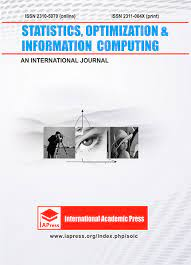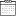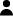# Necessary conditions to a fractional variational problem

• Home
• Necessary conditions to a fractional variational problem31 January 2022By CEE-M

In order to solve fractional variational problems, there exist two theorems of necessary conditions: an Euler-Lagrange equation which involves Caputo and Riemann-Liouville fractional derivatives, and other Euler-Lagrange equation that involves only Caputo derivatives. In this article, we make a comparison solving a particular fractional variational problem with both methods to obtain some conclusions about which method gives the optimal solution.